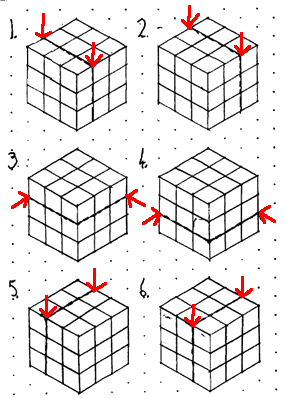#### You may also like### All in the Mind

Imagine you are suspending a cube from one vertex and allowing it to hang freely. What shape does the surface of the water make around the cube?### Painting Cubes

Imagine you have six different colours of paint. You paint a cube using a different colour for each of the six faces. How many different cubes can be painted using the same set of six colours?### Tic Tac Toe

In the game of Noughts and Crosses there are 8 distinct winning lines. How many distinct winning lines are there in a game played on a 3 by 3 by 3 board, with 27 cells?

# Cubist Cuts

##### Age 11 to 14 Challenge Level:

Jake, Ryo and Charlie from Moorfield Junior School have explained how to cut up a 3x3x3 cube using 6 cuts:

"We got a 3 by 3 cube and then we cut it 2 times to make 3 lots of 9 cubes. Then we piled all the cubes on top of each other. Then we took another 2 cuts to leave 9 towers of 3 cubes. Next we layed them next to each other. After that we took another 2 cuts to leave the 27 unit cubes."

Chris B, Elliot and Joseph, also from Moorfield Juniors, sent us a diagram to show where these cuts should be:Juliette noticed that it wouldn't be possible with fewer than 6 cuts:

"We need at least 6 cuts because we need one cut for each face of the small cube in the middle of the $3\times 3 \times 3$ cube."

Anthony noticed that, with a $4 \times 4 \times 4$ cube, we can use 6 cuts if we rearrange the cubes:

"First cut the cube in half down the middle, then stack the halves on top of each other (in an $8 \times 2 \times 4$ arrangement) and cut down the middle, to make four $4 \times 4$ slices each 1 unit thick. Then rearrange the cubes into the original arrangement and repeat the process in the other two directions. This will cut the cube into $1 \times 1 \times 1$ cubes. It cannot be done with fewer than 6 cuts because the cubes in the middle will each need at least one cut for each face"

The $n \times n \times n$ cube is a bit trickier. Try a few yourself before looking at this explanation.

First of all let's see how many cuts are needed to cut the cube into slices 1 unit deep. We can then do this in each of the three directions to cut the $n \times n \times n$ cube into unit cubes, and can multiply by three to find out how many cuts are needed in total.

For a cube with side length 3 or 4 units, we need 2 cuts, as Juliette and Anthony explained. For 5 units, we'll need an extra cut in each direction. To cut as efficiently as possible, we should use a method similar to Anthony's: first cut in half (or as close to in half as possible), then stack up the "halves" and repeat until we are left with "slices" 1 unit thick. We can then put the cube back together and repeat for the other two directions.

The general pattern is: for each doubling of $n$, we need an extra 3 cuts to cut an $n \times n \times n$ cube into $1 \times 1 \times 1$ cubes. This is shown in the table below.

 $n$ Number of cuts $1$ to $2$ $1 \times3$ $3$ to $4$ $2 \times3$ $5$ to $8$ $3 \times3$ $9$ to $16$ $4 \times3$ $(2^{k-1} + 1)$ to $2^k$ $k \times3$